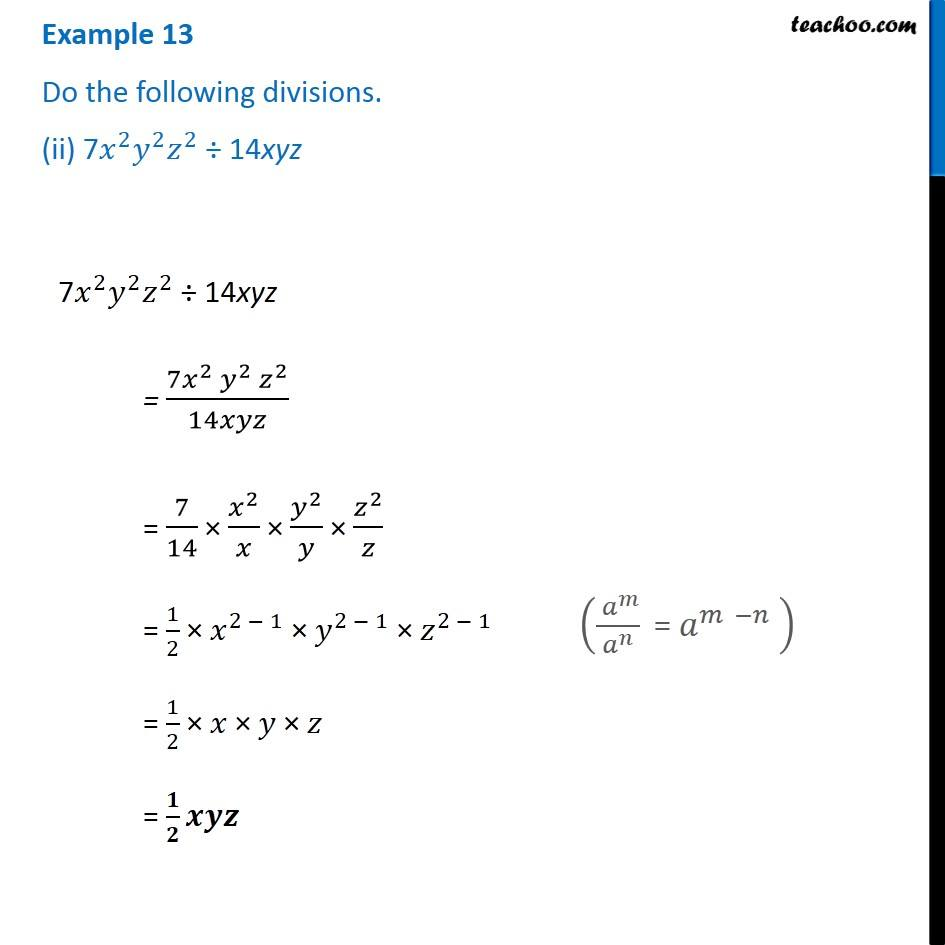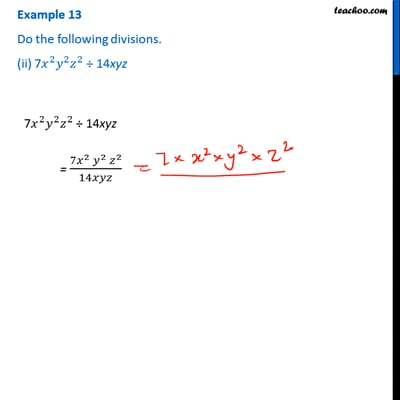Examples

Chapter 12 Class 8 Factorisation
Serial order wiseThis video is only available for Teachoo black users

Learn in your speed, with individual attention - Teachoo Maths 1-on-1 Class

### Transcript

Example 13 Do the following divisions. (ii) 7𝑥^2 𝑦^2 𝑧^2 ÷ 14xyz 7𝑥^2 𝑦^2 𝑧^2 ÷ 14xyz = (7𝑥^2 𝑦^2 𝑧^2)/14𝑥𝑦𝑧 = 7/14 × 𝑥^2/𝑥 × 𝑦^2/𝑦 × 𝑧^2/𝑧 = 1/2 × 𝑥^(2 − 1) × 𝑦^(2 − 1) × 𝑧^(2 − 1) = 1/2 × 𝑥 × 𝑦 × 𝑧 = 𝟏/𝟐 𝒙𝒚𝒛 (( 𝑎^𝑚)/𝑎^𝑛 " = " 𝑎^(𝑚 −𝑛) " " )